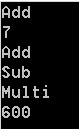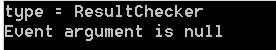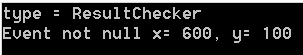# Inside Events in C #

A popular design pattern (a reusable solution for a recurring problems in software) in application development is that of publish-subscribe. Events in C# is the way to get notify about some thing interested. Delegates form the basis for the event system in .NET.

delegate is declared in C# in the following ways.

```public delegate int MyDelegate(int x, int y);
```

The above declaration declares MyDelegate can point to a method whose return value is integer and who takes 2 parameters. The following code shows how this cane be used.

```public class myApp
{
public delegate int MyDelegate ( int x, int y );

public static void Main ( )
{
MyDelegate del = new MyDelegate ( Add );
//Can be re written in the following way as well, compiler does the rest.
int result = del ( 3, 4 );
Console.WriteLine ( result ); // the output will be 7, not a big deal
}
public static int Add(int x, int y)
{
return x + y;
}
}
```

The above example is not a big deal. Let's rewrite the code that will use anonymous functions. The output of the program is as same as before but we have used anonymous function.

```public class myApp
{
public delegate int MyDelegate ( int x, int y );
public static void Main ( )
{
MyDelegate del = delegate ( int x, int y ) { return x + y; };
int result = del ( 3, 4 );
Console.WriteLine ( result );
}
}
```

We know delegate in C# can be chained. We can add many functions to the delegate variables as seen in the following example. All the anonymous methods are called one by one but the return value will be the return value of last executed function. In this example, the return value will be the result of multiplication.

```public class myApp
{
public delegate int MyDelegate ( int x, int y );
public static void Main ( )
{
MyDelegate del = delegate ( int x, int y ) { Console.WriteLine ( "Add" );  return x + y; };
int result = del ( 3, 4 );
Console.WriteLine ( result );
del += delegate ( int x, int y ) { Console.WriteLine ( "Sub" ); return x + y; };
del += delegate ( int x, int y ) { Console.WriteLine ( "Multi" ); return x * y; };
result = del ( 30, 20 );
Console.WriteLine ( result );
}
}
```

The output is shown in the following image.We can convert the previous example by using statement lambda. The output will be exactly same as previous example. Note that the variable x and y are int type, compilers infer the type.

```public class myApp
{
public delegate int MyDelegate ( int x, int y );
public static void Main ( )
{
MyDelegate del =  (  x,  y ) => { Console.WriteLine ( "Add" );  return x + y; };
int result = del ( 3, 4 );
Console.WriteLine ( result );
del +=  (  x,  y ) => { Console.WriteLine ( "Sub" ); return x + y; };
del +=  (  x,  y )  => { Console.WriteLine ( "Multi" ); return x * y; };
result = del ( 30, 20 );
Console.WriteLine ( result );
}
}
```

What is the use of these example so far related to event? Nothing! Let's we want to design or add a functionality to our example. We want to do addition, substraction and multiplication only when x and y are higher or equal than 100 we tell the client by firing the event. With our simple example we can check the result directly and print a message that the numbers are higher or equal than 100. It does not make very much sense. So let's re architect the whole a bit.

```public class ResultChecker
{
public delegate int MyDelegate ( int x, int y );
public MyDelegate OnSomeAction { get; set; }
public int RaiseEvent ( int x, int y )
{
int result = 0;
if (OnSomeAction != null)
{
result = OnSomeAction (x, y );
}
return result;
}
}
public class myApp
{
public static void Main ( )
{
ResultChecker checker = new ResultChecker ( );
checker.OnSomeAction +=  (  x,  y ) => { Console.WriteLine ( "Add" );  return x + y; };
checker.OnSomeAction += ( x, y ) => { Console.WriteLine ( "Sub" ); return x + y; };
checker.OnSomeAction += ( x, y ) => { Console.WriteLine ( "Multi" ); return x * y; };
Console.WriteLine ( checker.RaiseEvent ( 30, 20 ) );
}
}
```

This code almost same as previous except we have introduced new class ResultChecker. OnSomeAction is a property of delegate type. If you comment out 3 lines (start with checker.OnSomeAction) in Main method, the result will be 0, the reason OnSomeAction variable points to nothing. The output of the program is,

```Add
Sub
Multi
600
```

If there would be no subscribers to an event, the OnChange property would be null. This is why the RaiseEvent method checks to see whether OnChange is not null. This sounds doing some work related to event but there are some problems here.
1. Change the code in Main method like this and see that Add and Sub are not printed, meaning those are not called.

```checker.OnSomeAction = ( x, y ) => { Console.WriteLine ( "Multi" ); return x * y; };
```

2. Violation of object oriented concept, anybody can fire an event by calling

`checker.RaiseEvent ( 30, 20 ); `

These 2 problems are overcome by using event keyword. If we try to assign something to OnSomeAction it will generates an error. Also another issues, if we try to raise the event directly it will complains. Previously we could call directly OnSomeAction(30, 20) but now it is not possible anymore because of the event keyword. In the following example we call the methods only if it is higher or equal to 100.

```public class ResultChecker
{
public delegate int MyDelegate ( int x, int y );
public event MyDelegate OnSomeAction;
public int RaiseEvent ( int x, int y )
{
int result = 0;
if (OnSomeAction != null)
{
if (x >= 100 && y >= 100)
{
result = OnSomeAction ( x, y );
}
}
return result;
}
}
public class myApp
{
public static void Main ( )
{
ResultChecker checker = new ResultChecker ( );
checker.OnSomeAction += ( x, y ) => { Console.WriteLine ( "Add" ); return x + y; };
checker.OnSomeAction += ( x, y ) => { Console.WriteLine ( "Sub" ); return x + y; };
checker.OnSomeAction += ( x, y ) => { Console.WriteLine ( "Multi" ); return x * y; };
checker.OnSomeAction += checker_OnSomeAction;
Console.WriteLine ( checker.RaiseEvent ( 60, 10 ) ); // does not call method
Console.WriteLine ( checker.RaiseEvent ( 600, 100 ) );
}
static int checker_OnSomeAction ( int x, int y )
{
Console.WriteLine ( "Div" ); return (int)(x / y);
}
}
```

Final code after some modification

```public class ResultChecker
{
public delegate void MyDelegate ( int x, int y );
public event MyDelegate OnSomeAction;
public void CallMethod ( int x, int y )
{
if (OnSomeAction != null)
{
if (x >= 100 && y >= 100)
{
OnSomeAction ( x, y );
}
}
}
}
public class myApp
{
public static void Main ( )
{
ResultChecker checker = new ResultChecker ( );
checker.OnSomeAction += checker_OnSomeAction;
checker.CallMethod ( 60, 10 ); // does not call
checker.CallMethod ( 600, 100 );
}
static void checker_OnSomeAction ( int x, int y )
{
Console.WriteLine ( "Div x = {0}, y = {1}" , x, y);
Console.WriteLine (  (int)(x / y));
}
}
```

Following listing uses some special syntax to initialize the event to an empty delegate. This way, we can remove the null check around raising the event because we can be certain that the event is never null. Outside users of our class can’t set the event to null; only members of our class can. As long as none of our other class members sets the event to null, we can safely assume that it will always have a value.

```public class ResultChecker
{
public delegate void MyDelegate ( int x, int y );
public event MyDelegate OnSomeAction = delegate { };
public void CallMethod ( int x, int y )
{
if (x >= 100 && y >= 100)
{
OnSomeAction ( x, y );
}
}
}
public class myApp
{
public static void Main ( )
{
ResultChecker checker = new ResultChecker ( );
//Comment the below line and notice that the event is not fired! though there is no null check
checker.OnSomeAction += checker_OnSomeAction;
checker.CallMethod ( 600, 100 );
}
static void checker_OnSomeAction ( int x, int y )
{
Console.WriteLine ( "Div x = {0}, y = {1}" , x, y);
Console.WriteLine (  (int)(x / y));
}
}
```

So far it looks the event we have developed it works but this is not enough to satisfy the .NET naming convention. We can't declare our own delegate, we need to follow the signature specified by framework.

`public delegate void EventHandler(object sender, EventArgs e);`

or the following

`public delegate void EventHandler( Object sender, TEventArgs e) `

The sender is by convention the object that raised the event (or null if it comes from a static method). EventArgs provide extra information about the event. Let change the code according to specification.

```public class ResultChecker
{
public delegate void EventHandler ( object sender, EventArgs e );
public event EventHandler OnSomeAction = delegate { };
public void CallMethod ( int x, int y )
{
if (x >= 100 && y >= 100)
{
OnSomeAction ( this, EventArgs.Empty );
}
}
}
public class myApp
{
public static void Main ( )
{
ResultChecker checker = new ResultChecker ( );
checker.OnSomeAction += checker_OnSomeAction;
checker.CallMethod ( 600, 100 );
}
static void checker_OnSomeAction ( object sender, EventArgs e )
{
Type type = sender.GetType ( );
Console.WriteLine ( "type = {0}", type.ToString ( ) );
if (e == EventArgs.Empty)
{
Console.WriteLine ( "Event argument is null" );
}
else
{
Console.WriteLine ( "Event argument is not null" );
}
}
}
```

Output of the above program is shown in the following image. Since we pass the empty event, we can't pass anything in the argument.Now we can create class for custom event argument so we can pass some useful information. We want to pass back the 2 numbers we passed to the delegate. NumberArgs is derived from EventArgs.

```public class NumberArgs : EventArgs
{
public NumberArgs (int _x, int _y)
{
x= _x;
y= _y;
}
public int x{ get; set; }
public int y{ get; set; }
```

We pass the NumberArgs  in the following way,

`OnSomeAction ( this,  new NumberArgs(x,y) ); `

Now the updated code

```public class ResultChecker
{
public delegate void EventHandler ( object sender, EventArgs e );
public event EventHandler OnSomeAction = delegate { };
public void CallMethod ( int x, int y )
{
if (x >= 100 && y >= 100)
{
OnSomeAction ( this,  new NumberArgs(x,y) );
}
}
}

public class NumberArgs : EventArgs
{
public NumberArgs ( int _x, int _y )
{
x = _x;
y = _y;
}
public int x { get; set; }
public int y { get; set; }
}

public class myApp
{
public static void Main ( )
{
ResultChecker checker = new ResultChecker ( );
checker.OnSomeAction += checker_OnSomeAction;
checker.CallMethod ( 600, 100 );
}
static void checker_OnSomeAction ( object sender, EventArgs e )
{
Type type = sender.GetType ( );
Console.WriteLine ( "type = {0}", type.ToString ( ) );
if (e == EventArgs.Empty)
{
Console.WriteLine ( "Event argument is null" );
}
else
{
NumberArgs arg = (NumberArgs)e;
Console.WriteLine ( "Event not null x= {0}, y= {1}", arg.x, arg.y );
}
}
}
```

Output of above code is shows in the following figure.Final code so far, we added statement lamda back with a static method.

```
public class ResultChecker
{
public event EventHandler OnSomeAction = delegate { };
public void CallMethod ( int x, int y )
{
if (x >= 100 && y >= 100)
{
OnSomeAction ( this, new NumberArgs ( x, y ) );
}
}
}

public class NumberArgs : EventArgs
{
public NumberArgs ( int _x, int _y )
{
x = _x;
y = _y;
}
public int x { get; set; }
public int y { get; set; }
}

public class myApp
{
public static void Main ( )
{
ResultChecker checker = new ResultChecker ( );

checker.OnSomeAction += ( object sender, NumberArgs e ) =>
{
Type type = sender.GetType ( );
Console.WriteLine ( "type = {0}", type.ToString ( ) );
if (e == EventArgs.Empty)
{
Console.WriteLine ( "Event argument is null" );
}
else
{
NumberArgs arg = (NumberArgs)e;
Console.WriteLine ( "Event not null x= {0}, y= {1}", arg.x, arg.y );
}
};

checker.OnSomeAction += checker_OnSomeAction;
checker.CallMethod ( 600, 100 );
}
static void checker_OnSomeAction ( object sender, EventArgs e )
{
Type type = sender.GetType ( );
Console.WriteLine ( "type = {0}", type.ToString ( ) );
if (e == EventArgs.Empty)
{
Console.WriteLine ( "Event argument is null" );
}
else
{
NumberArgs arg = (NumberArgs)e;
Console.WriteLine ( "Event not null x= {0}, y= {1}", arg.x, arg.y );
}
}
}
```

This site is updated and maintained by Mahbub Rahman.
Updated on 07/20/2015